## Example Questions

### Example Question #1 : Rectangles

Given Rectangle ABCD.

Quantity A: The length of diagonal AC times the length of diagonal BD

Quantity B: The square of half of ABCD's perimeter

Quantity A is greater.

The relationship cannot be determined from the information given.

Quantity B is greater.

The two quantities are equal.

Quantity B is greater.

Explanation:

Suppose ABCD has sides a and b.

The length of one of ABCD's diagonals is given by a2+ b2 = c2, where c is one of the diagonals.

Note that both diagonals are of the same length.

Quantity A: The length of diagonal AC times the length of diagonal BD

This is c * c = c2.

Quantity A = c2 = a2+ b2

Now for Quantity B, remember that the perimeter of a rectangle with sides a and b is Perimeter = 2(a + b).

Half of Perimeter = (a + b)

Square Half of Perimeter = (a + b)2

Use FOIL: (a + b)2 = a2+ 2ab + b2

Quantity B = (a + b)2 = a2+ 2ab + b2

The question is asking us to compare a2+ b2 with a2+ 2ab + b2.

Note that as long as a and b are positive numbers (in this case a and b are dimensions of a rectangle, so they must be positive), the second quantity will be greater.

### Example Question #5 : Rectangles

If rectanglehas a perimeter of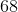, and the longer edge is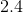times longer than the shorter edge, then how long is the diagonal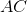?Explanation:

Lets call our longer side L and our shorter side W.

If the perimeter is equal to 68, then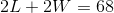.

We also have that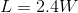.

If we then plug this into our equation for perimeter, we get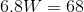.

Therefore,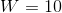and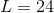. Using the Pythagorean Theorem, we have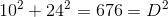so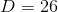.

Tired of practice problems?

Try live online GRE Math prep today.